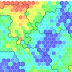# The Numpy, Scipy, Pandas, and Matplotlib stack: prep for deep learning, machine learning, and artificial intelligence

Created by Lazy Programmer Inc.
Last updated 8/2017
English
What Will I Learn?
• Understand and code using the Numpy stack
• Make use of Numpy, Scipy, Matplotlib, and Pandas to implement numerical algorithms
Requirements
• Understand linear algebra and the Gaussian distribution
• Be comfortable with coding in Python
• You should already know “why” things like a dot product, matrix inversion, and Gaussian probability distributions are useful and what they can be used for
Description
Welcome! This is Deep Learning, Machine Learning, and Data Science Prerequisites: The Numpy Stack in Python.
One question or concern I get a lot is that people want to learn deep learning and data science, so they take these courses, but they get left behind because they don’t know enough about the Numpy stack in order to turn those concepts into code.
Even if I write the code in full, if you don’t know Numpy, then it’s still very hard to read.
This course is designed to remove that obstacle – to show you how to do things in the Numpy stack that are frequently needed in deep learning and data science.
So what are those things?
Numpy. This forms the basis for everything else.  The central object in Numpy is the Numpy array, on which you can do various operations.
The key is that a Numpy array isn’t just a regular array you’d see in a language like Java or C++, but instead is like a mathematical object like a vector or a matrix.
That means you can do vector and matrix operations like addition, subtraction, and multiplication.
The most important aspect of Numpy arrays is that they are optimized for speed. So we’re going to do a demo where I prove to you that using a Numpy vectorized operation is faster than using a Python list.
Then we’ll look at some more complicated matrix operations, like products, inverses, determinants, and solving linear systems.
Pandas. Pandas is great because it does a lot of things under the hood, which makes your life easier because you then don’t need to code those things manually.
Pandas makes working with datasets a lot like R, if you’re familiar with R.
The central object in R and Pandas is the DataFrame.
We’ll look at how much easier it is to load a dataset using Pandas vs. trying to do it manually.
Then we’ll look at some dataframe operations, like filtering by column, filtering by row, the apply function, and joins, which look a lot like SQL joins.
So if you have an SQL background and you like working with tables then Pandas will be a great next thing to learn about.
Since Pandas teaches us how to load data, the next step will be looking at the data. For that we will use Matplotlib.
In this section we’ll go over some common plots, namely the line chart, scatter plot, and histogram.
We’ll also look at how to show images using Matplotlib.
99% of the time, you’ll be using some form of the above plots.
Scipy.
I like to think of Scipy as an addon library to Numpy.
Whereas Numpy provides basic building blocks, like vectors, matrices, and operations on them, Scipy uses those general building blocks to do specific things.
For example, Scipy can do many common statistics calculations, including getting the PDF value, the CDF value, sampling from a distribution, and statistical testing.
It has signal processing tools so it can do things like convolution and the Fourier transform.
In sum:
If you’ve taken a deep learning or machine learning course, and you understand the theory, and you can see the code, but you can’t make the connection between how to turn those algorithms into actual running code, this course is for you.
All the code for this course can be downloaded from my github: /lazyprogrammer/machine_learning_examples
In the directory: numpy_class
Make sure you always “git pull” so you have the latest version!
HARD PREREQUISITES / KNOWLEDGE YOU ARE ASSUMED TO HAVE:
• linear algebra
• probability
• Python coding: if/else, loops, lists, dicts, sets
• you should already know “why” things like a dot product, matrix inversion, and Gaussian probability distributions are useful and what they can be used for
TIPS (for getting through the course):
• Watch it at 2x.
• Take handwritten notes. This will drastically increase your ability to retain the information.
• Ask lots of questions on the discussion board. The more the better!
• Realize that most exercises will take you days or weeks to complete.
USEFUL COURSE ORDERING:
• (The Numpy Stack in Python)
• Linear Regression in Python
• Logistic Regression in Python
• (Supervised Machine Learning in Python)
• Deep Learning in Python
• Practical Deep Learning in Theano and TensorFlow
• Convolutional Neural Networks in Python
• (Easy NLP)
• (Cluster Analysis and Unsupervised Machine Learning)
• Unsupervised Deep Learning
• (Hidden Markov Models)
• Recurrent Neural Networks in Python
• Natural Language Processing with Deep Learning in Python
Who is the target audience?
• Students and professionals with little Numpy experience who plan to learn deep learning and machine learning later
• Students and professionals who have tried machine learning and data science but are having trouble putting the ideas down in code
Credit: Michael Scofield <<< Thank you! ?
Size: 243.35M

DEEP LEARNING PREREQUISITES: THE NUMPY STACK IN PYTHONReviewed by Syasu on July 11, 2018 Rating: 5